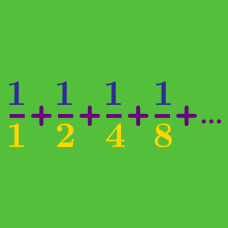Calculus

# Finding the nth Term

Guess the next term of the following sequence:

$2, \quad 3, \quad 5, \quad 8, \quad 12, \quad 17, \quad \square$

What is the $6 ^{th}$ term of the sequence $\{ n^2 + 2 n + 8 \}_{n=1}^\infty?$

The first $n$ terms of a sequence sum to $n(n+2)(2n+1)$. What is the value of the $6 ^{th}$ term?

The sequence $\{a_n\}$ satisfies $a_1=1$ and $a_{n+1}=11 ^n a_n \text{ for } n \geq 1.$

For what value of $k$ do we have $a_k=11 ^{276}$?

If $\{a_n\}$ is an arithmetic progression with $a_{11}=133$ and $a_{23}=313$, what is $a_{50}$?

×